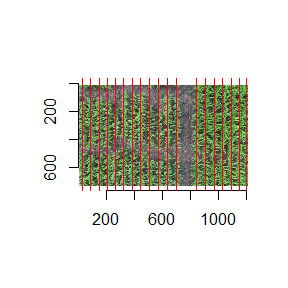# CropDetectR

#### 12 September, 2019

library(CropDetectR)
library(imager)
library(ggplot2)
library(dplyr)
library(reshape2)
library(EBImage)
library(stats)

# Grayscale using ExG

Loading the image using the EBImage loading function then change it to imager format to prepare it for the ExG process.

img <- readImage('https://raw.githubusercontent.com/niconaut/CropDetectR/master/images/before_rows.JPG')
img <- as.cimg(img[1:length(img)], dim = c(dim(img), dim(img), 1, dim(img)))

Notice how the crops and some of the smaller weeds range from dark gray to white. This is due to the ExG method of putting all the pixel colors on a scale from non-organic material to definatly plant material.

img_grayscale <- make_ExG(img)

plot(img)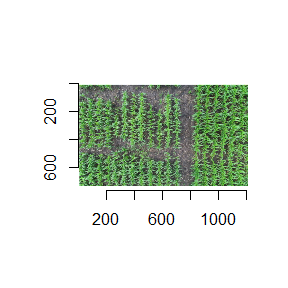plot(img_grayscale)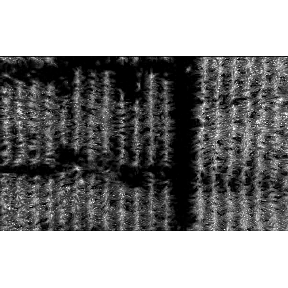# Otsu Transformation

Using the Otsu method of image thresholding, we are able to capture only the plant material as white (value of 1) and the rest of the non-organic material as black (value of 0). This will be helpful for the next steps because a binary image is needed.

black_white <- make_bw(img_grayscale)

plot(black_white)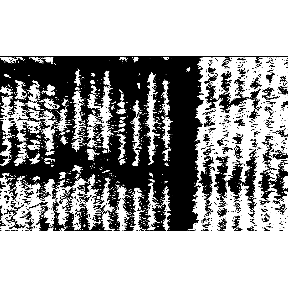# Morphology

Through the use of morphology we have only kept the important parts of the crop rows and erased all the extrenuous leaves and weeds. By using opening and closing techniques we were able to have a simpler, more concentrated image of our crop rows.

img_blob <- blobify(black_white, 3) # min input value of 1

plot(img_blob)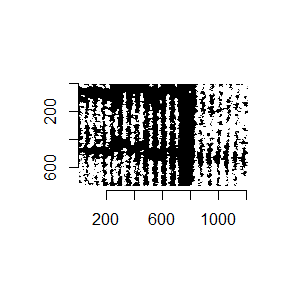# Rotating the Image

Performing simple image rotations will allow us to find out the best degree of rotation to get the crop rows vertical. In this example we are rotating the image every 45 degrees to check if there is a better angle other than its base. It is important that they are vertical for the next few steps.

oldpar <- par(mfrow = c(1,2))

par(mar = rep(2,4))
rotations_list <- rotations(img_blob, 90)

plot(rotations_list)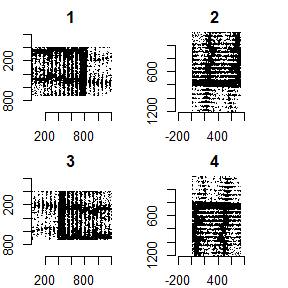par(oldpar)

# Smoothening the images

Making the images smoother is necessary when we are trying to find true local minimas and maximas. If the image is very sparatic and jagged, identifying the locals will be a tough challenge since there is a lot of up and down change. As we see in the smoothed image, there are only a few locals. In the next steps, we can use a ratio to ignore the smaller maximas and minimas, leaving us with only the very pronounced ones.

jagged <- as.data.frame(rotations_list) %>%
select(x,y,value) %>%
group_by(x) %>%
summarise(row_threshold = mean(value))

jagged_array <- as.array(jagged\$row_threshold)

plot(jagged_array, type = 'l')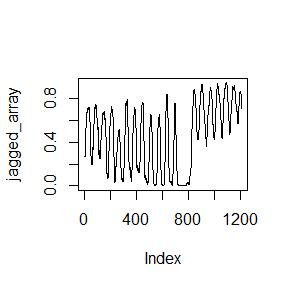smooth <- smoothing(rotations_list, 0.25)

plot(smooth, type = 'l')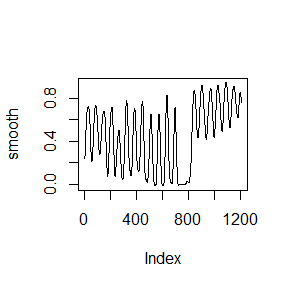# Finding the best rotation

To find the best rotation we rely on the smoothed arrays as seen above. Now that our array has prominent maximas and minimas we can use an arbitrary ratio to count the number of good ratios in each array. This is done by comparing neighbor maximas and minimas y-value ratios. If there is a large ratio, that means the contrast between the crop row and its surrounding ‘dirt space’ is high; this implies a crop row present. If the ratio is low, it is most likely weeds or random noise. While we do not need to use this function as a stand alone, it is still beneficial to see how the best image is determined because it is apart of the final step.

best_img <- best_rotation(rotations_list, 0.5, 0.25)

paste("The best rotation was rotation ", best_img - 1, ".", sep = "")
#>  "The best rotation was rotation 0."

plot(smoothing(rotations_list[best_img], 0.25), type = 'l')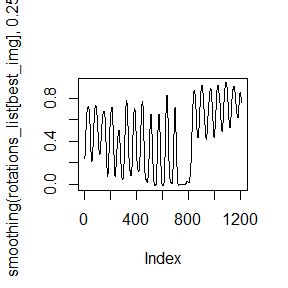# Finding the crop row lines

This final step uses the best image we previously found, as well as the maximas and minimas that passed the good ratio test discussed earlier. When finding the crop row centers, we are only concerned with the maximas since they are the peaks. This correlates to the center of the crop rows. The minimas are the center of the spaces between the crops, which are not a concern to us. As seen previously, we used a ratio to find the amount of good ratios for each image. With this final step, we use that known best image but apply a much more lienent ratio to it. This will capture less pronounced crop rows that may have not passed the good ratio test. While this number is also arbitrary, ~ 0.05 works well for many crop row images.

picture_list <- rotations_list

crop_rows <- crop_row_finder(rotations_list, 0.5, 0.05, 0.25)

plot(smoothing(rotations_list[best_img], 0.25), type = 'l')
abline(v = crop_rows, col = "red")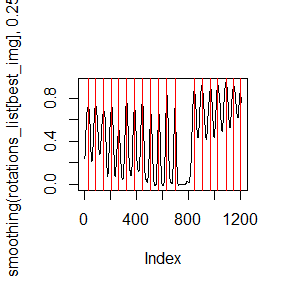plot(img)
abline(v = crop_rows, col = "red", lwd = 1.5)